# Is Sodium hydroxide (NaOH) an acid or base? Strong vs Weak - Conjugate acid

Home  > Chemistry Article > Is NaOH an acid or base?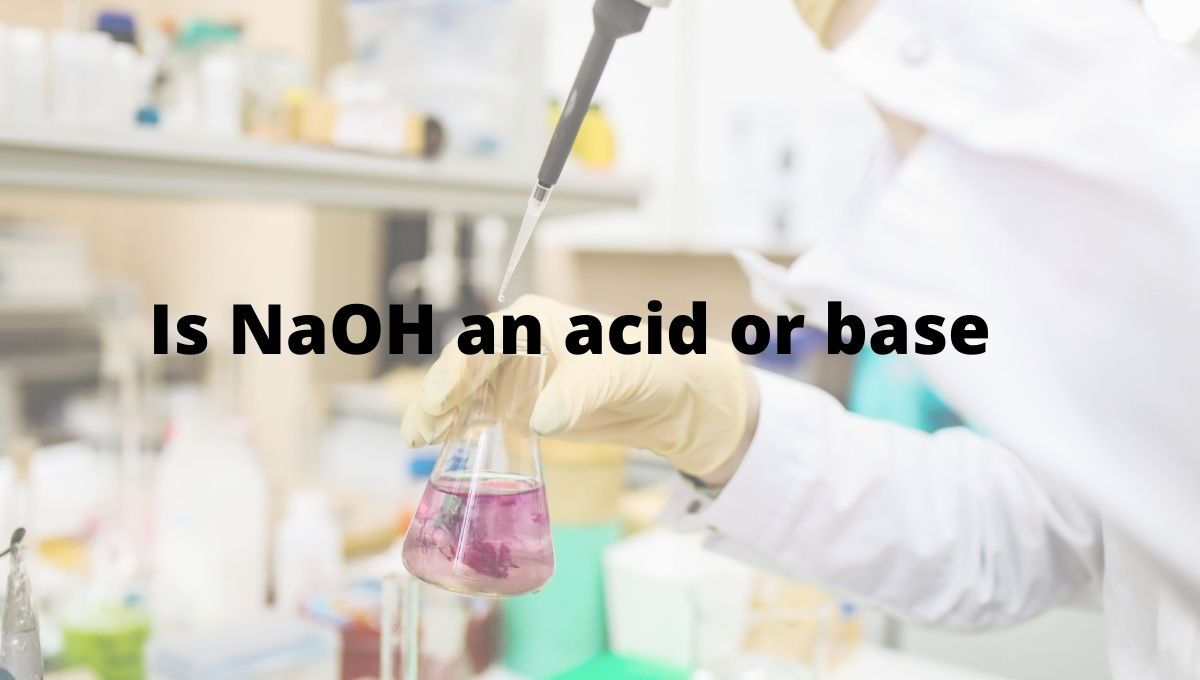Sodium hydroxide is also known as soda-lime has the chemical formula NaOH. It is made up of Na+ cation and OH- anion. It is mainly used for manufacturing soaps, detergents, and paper.

In this article, we will discuss Is Sodium hydroxide (NaOH) an acid or base? Is it strong or weak? Its conjugate acid-base pairs, etc.

So, Is NaOH an acid or base? NaOH is the base because when dissolved in water, it gives two ions Na+ and OH. And any compound that releases OH ion in an aqueous solution is Base in nature. It has a pH value of 12. This pH value can reach up to 13-14 in the pure concentration.

NaOH is one of the important bases uses in various industrial applications.

 Name of Molecule Sodium hydroxide Chemical formula NaOH pH value 12 – 14 Conjugate acid Na+ Nature Strong base Basicity (pKb) 0.2 Solubility in water miscible
Page Contents

## Why NaOH is base?

A base is defined as a proton acceptor or electron-pair donor. When NaOH dissolve in water, it split into two ions Na+ and OH

As NaOH dissociates into Na+ and OH, this OH ion accepts the proton (H+) to form water.

⇒ NaOH(aq) → Na+ (aq) + OH– (aq)

Theoretically, we have three theories to know whether NaOH is acid or base.

(a). Arrhenius theory

(b). Bronsted-Lowry theory

(c). Lewis theory

Let’s check whether NaOH acid or base according to these theories.

(1). Arrhenius theory:

According to the Arrhenius theory, the compound is said to be base when it produces OH ions through ionization or through dissociation in water and increases the concentration of OH ions in an aqueous solution.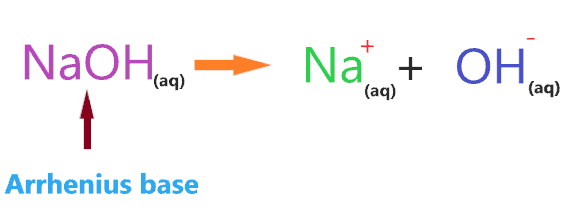Clearly, when NaOH is dissolved in an aqueous solution it produces OH ions, hence, NaOH is an Arrhenius base.

(2). Bronsted-Lowry theory:

According to the Bronsted-Lowry theory, a compound is said to be base when it accepts the proton from other compounds. Or you can say proton acceptor compounds are classified as Bronsted-Lowry base.

Take an example for knowing whether NaOH base or acid according to the Bronsted-lowry concept-

Consider the reaction of NaOH with HCl.According to the above reaction, NaOH has two ions Na+ and OH, when they react with HCl then OH accepts H+ from HCl to form H2O.

So, NaOH ion accepting the proton or hydrogen ion from another compound, and according to this theory, NaOH will act as a Bronsted-Lowry base since it accepting the proton and HCl will act as a Bronsted-Lowry acid since it donating the proton.

(3). Lewis theory:

Here comes the most important concept of acid and base. According to the lewis theory, a compound is said to be acid when it accepts the pair of electrons and a compound is said to be base when it donates the pair of electrons.

What does it mean?

It means Lewis acid is a compound that has a deficiency of electrons that’s why they are electron-pair acceptors and lewis’s base is a compound that is rich in electrons so, they are electron-pair donors.

Lewis acid → electron pair acceptor

Lewis base → electron pair donator

As NaOH contains OH ion that has three lone pairs of electrons and it can easily donate these pairs of electrons to form a covalent bond with another compound acceptor.

So, we can say NaOH is lewis’s base also.

## Is Sodium hydroxide(NaOH) a strong or weak base?

To know whether Sodium hydroxide (NaOH) is a strong base or a weak base, you must know the basic difference between a strong base or a weak base.

Strong base: A compound is a strong base when it completely dissociates in an aqueous solution and liberates a large number of hydroxide ions. All moles of the base dissociate to yield hydroxide ion(OH) and no undissociated base should be left in the solution.

Example: Barium hydroxide (Ba(OH)2), Calcium hydroxide (Ca(OH)2), Lithium hydroxide (LiOH), Potassium hydroxide (KOH), etc.

Weak base: A compound is a weak base when it partially or not completely dissociate in an aqueous solution which means not all moles of the base dissociate in a solution to yield OH ion, and at equilibrium, both undissociated base and their ionized product present in the solution.

Example- Ammonia (NH3), Ammonia hydroxide (NH4OH), Methylamine ( CH3NH2), etc.

 Strong base Weak base They ionize completely. They do not completely ionize. They are highly reactive. They are less reactive compare to a strong base. The value of pH lies between 10 to 14. The value of pH lies between 7 to 10. They have a high equilibrium constant. They have a less equilibrium constant. They are good electrolytes. They are not so good electrolytes compare to a strong base. Example – NaOH, KOH, LiOH, etc. Example – CH3NH2, NH3, NH4OH, etc.

So, Is Sodium hydroxide (NaOH) a strong base or a weak base? NaOH is the strong base because it completely dissociates in an aqueous solution to give OH ion and no moles of it remain undissociated inside the solution. And the amount of OH ions in an aqueous solution is very high and we know OH ions have a tendency to accept the proton.

So, more proton acceptors present in the solution ultimately make the NaOH a strong base.As you see in the above aqueous solution, when NaOH is dissolved in water, it is completely ionized into the ions(Na+ and OH). No undissociated molecule(NaOH) present in the solution, only ionized ions present everywhere in the solution.

The single arrow used in the above reaction shows that only a forward reaction takes place that means, once the NaOH molecule breaks into the ions, these ions will not reform with each other to form NaOH again.

As Na+ is a very weak conjugate acid of NaOH, hence it has no ability to react with either OH ion or with water molecules ions. Thus, only splitting ions(Na+ and OH) remain in the solution.

Or you can also assume the Na+ as a spectator ion because it is almost useless in solution, it has no effect on the pH value of the solution.

“A spectator ion is an ion that does not take part in the chemical reaction and is found in solution both before and after the reaction.”

Hence, a large number of hydroxide ions present in the aqueous solution of NaOH, steadily increase the pH value and rises the effect of the basic in solution. And all these make sodium hydroxide a strong base in nature.

Also, the base dissociation constant value(Kb) for NaOH is approx 1020, and that’s huge.

An base dissociation constant (Kb) is a quantitative measure of the strength of an base in solution.

⇒ If the value of the dissociation constant of the base is greater than 1 (Kb >> 1), then the nature of the compound is a strong base.

⇒ If Kb << 1, then the nature of the compound is a weak base.

So, the higher the value of the base dissociation constant, the larger is the strength of a base in solution.

Here’s the list of some common strong/weak acids and bases.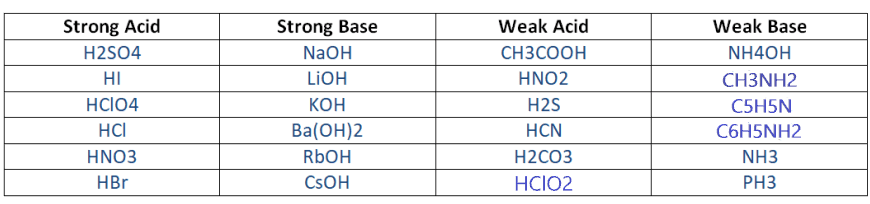## Why Sodium hydroxide(NaOH) is not an acid?

Acid is a compound that donates H+ ions when dissolving them in an aqueous solution. It means the acid is the proton donator.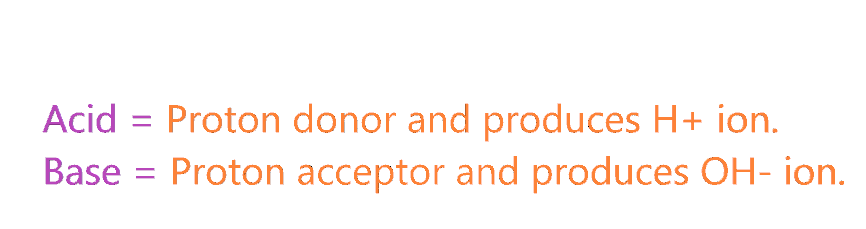So, Is NaOH is an acid? NaOH is not an acid because when it dissolves in an aqueous solution, it furnishes a large amount of OH ions. And OH ion is a good acceptor of the proton, which means NaOH ions are proton acceptors, not a proton donator.

NaOH + (aq) → Na+ (aq) + OH– (aq)

We have two theories given by Arrhenius and Bronsted-Lowry to check why NaOH is not acid?

According to the Arrhenius theory, any compound is said to be acid when it liberates H+ ion in water or aqueous solution. And we know NaOH in an aqueous solution only produces OH ion. So, NaOH is not an Arrhenius acid.

According to the Bronsted-lowry theory, any compound is said to be acid when it has a tendency to donate the proton to other compounds. Let’s take an example to understand this-

NaOH(aq) + HCl(aq) → NaCl(aq) +H2O(l)

When NaOH reacts with a strong acid (HCl), the OH ion accepts H+ from HCl to produce H2O, which means here NaOH act as a proton acceptor.

So, here, NaOH is not a Bronsted-lowry acid since it is accepting the proton.

## Why NaOH act as a Lewis base?

Lewis’s theory is a very important acid-base theory to check whether a compound (NaOH) acid or base?

According to the Lewis theory, a compound is said to be acid when it accepts the pair of electrons and a compound is said to be base when it donates the pair of electrons.

Lewis acid → lone pair acceptor

Lewis base → lone pair donator

Now, why does NaOH act as a Lewis base? To know this first we should know what type of compound is Lewis acid and Lewis base.

Lewis acid: They are electron-loving compounds and are also known as electrophilic compounds that accept the electron pair from Lewis bases.

Some examples of Lewis acids that can accept the pair of electrons from another species.

1. The molecules or ions with an incomplete octet of electrons. Example – BF3, BCl3, AlF3, etc.
2. The molecule that central atom has an empty d-orbital. Example- SiCl4 (1s22s22p63s23p3d0).
3. The compounds that the central atom formed multiple bonds with the adjacent atoms. Example – SiO2 (O = Si = O), CO2, SO2, etc.
4. Simple cations like H+, Na+, Mg+2, Al+3, etc. are lewis acids since they are able to accept the electrons. ( Exception NH4+, PH4+ are not a lewis acid)

Lewis base: They are electron-rich species and also known as nucleophile compounds that donate the electron pairs to the Lewis acids.

Some examples of Lewis bases that can donate the pair of electrons to other species.

1. The negative ion such as H, OH, F, etc.
2. Species that have lone pair of electrons. Example – NH3, H2O, CH3, etc.

Let’s take an example to understand why NaOH act as Lewis base?

⇒ NaOH + HCl → NaCl + H2OAs we know, In the HCl molecule, the chlorine atom is more electronegative than the hydrogen atom, therefore, more electrons will be attracted to the side of the chlorine atom, which will induce a negative charge on the chlorine atom and a slightly positive charge on the hydrogen atom.

Now, in the NaOH compound, the oxygen atom has lone pair that is attracted towards the slightly positive hydrogen atom in the HCl molecule.

As the lone pair on the oxygen atom approaches the hydrogen atom, the electrons in the HCl bond are repelled still further towards the chlorine atom.

And in this process, a coordinate bond is formed between the hydrogen and hydroxyl group, and the chlorine breaks away.

Therefore, the HCl molecule will act as a Lewis acid as it accepting the pair of electrons from NaOH and, in this process, it breaks up. [Source google: Lewis acid & base]

So, HCl accepts the lone pair of the electron, therefore, it is Lewis acid and NaOH donates the lone pair of the electron, therefore, it is Lewis base.

## What is the conjugate acid of NaOH?

In technical terms, Compounds differentiated from each other by a single proton(H+) are said to be Conjugate acid-base pairs.

In simple terms, when the proton is removed from parent acid then the compound is formed which is called the conjugate base of that acid and when the proton is added to the parent base then the compound is formed which is called conjugate acid of that base.

Concept of Conjugate acid-base pair-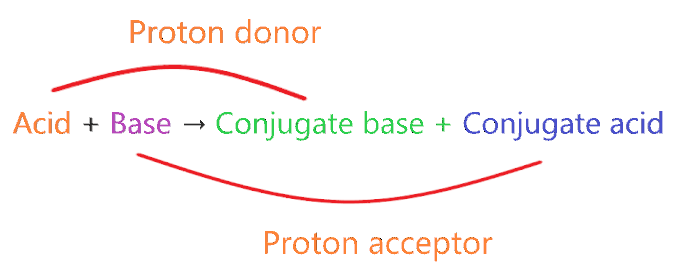• A very weak acid forms the strong conjugate base.
• A very weak base forms strong conjugate acid.
• A very strong acid forms the weak conjugate base.
• A very strong base forms weak conjugate acid.

⇒ Very weak means it doesn’t act as acid or base when dissolved in an aqueous solution.

⇒ Very strong means, acid or base ionizes 100% when dissolved in an aqueous solution.

Example 1: NH3 + H+ → NH4+

Here in this reaction, NH3 is a weak base and we know a weak base always forms a conjugate acid(not necessarily the strong one).

So, NH4is the conjugate acid of NH3.

Example 2: HCl → H+ Cl

In this reaction, HCl is a very strong acid and we know very strong acid always forms the weak conjugate base by donating one proton.

So, Cl is the weak conjugate base of HCl.

Example 3: CH3COOH + H2O → CH3COO + H3O+

Here in this reaction, that donates the proton to form CH3COO, which means CH3COO– is the conjugate base of CH3COOH.

H2O behaves as the base because it accepts the proton from CH3COOH to form H3O+, which means H3O+ is the conjugate acid of H2O.

⇒ The Conjugate acid of NaOH is Na+. [Check here]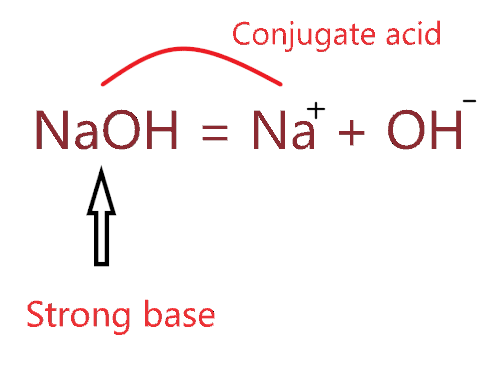So, only OH ion is left and conjugate acid is formed when H+ is added.

Therefore, OH + H+ = H2O is the conjugate acid of the hydroxide ion(OH).

## How to know if NaOH is acid or base practically?

Till now we learn how to know if compound acid or not theoretically. To know practically, one of the easiest ways to use litmus paper.

Litmus is a water-soluble mixture of different dyes extracted from lichens. It is often absorbed onto filter paper to produce one of the oldest forms of pH indicator, used to test materials for acidity. [Wiki]

Note: When Red litmus paper turns blue then the compound is said to be base. And when blue litmus paper turns red then the compound is said to be acidic.

When NaOH is contacted with red litmus paper then litmus paper turns into blue color. So, we can say NaOH is the base.

## Is NaOH alkali or not?

Alkali is a strong base that produces hydroxide ions when it is dissolved in water. All soluble hydroxides like lithium, cesium, sodium, potassium, etc. are alkali metals.

An alkali is said to be strongest when it produces almost all OH ions when it is dissolved in water. As NaOH molecule, when dissolved in water produce almost all OH ions that ultimately make it strong alkali.

## Uses of Sodium hydroxide

• NaOH is used as a neutron moderator.
• It is used to prevent the segregation of sand and cement.
• It is used for detecting carbon monoxide poisoning.
• It is used to purify water by increasing its pH value.
• It is used as a cleansing agent.
• It is widely used in pulpwood for making paper and fibers.
• It’s used for making textile fibers.
• The most widespread use of sodium hydroxide is done for making soaps and detergent because it is cheaper and easy to use.

## Conclusion

I hope you must enjoy this article on Is Sodium hydroxide(NaOH) an acid or base? And I think this article clears all of the doubt you have over Why NaOH is base, why it’s not an acid, is its a strong base or not, etc. Still, if you have a doubt regarding this then you must clear it by asking in a comment box. Lastly, I am giving you a short overview of this article.

• Is NaOH an acid or base? Sodium hydroxide (NaOH) is the base since it releases OH ions on dissolving in an aqueous solution.
• NaOH is the strong base because it completely dissociates in an aqueous solution and produces a higher amount of OH ions.
• We have three theories to check whether NaOH base or acid. (a). Arrhenius, Bronsted-Lowry, Lewis acid-base theory, etc.
• NaOH act as a Lewis base as it has an OH ion which can donate the electron pair to another compound acceptor.
• The base dissociation constant value for NaOH is approximately 1020.
##### Subscribe to Blog via Email

Join 2 other subscribers

Share it...